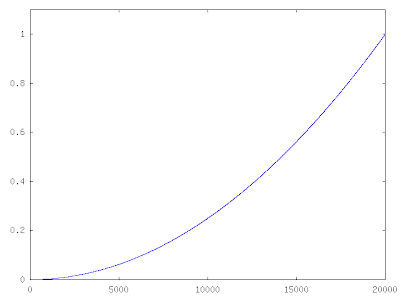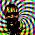## Thursday, October 23, 2008

### Is Octave Slow?

I'm not going to talk about "computer time" vs. "programmer time"; though that's probably one of the most important considerations most of the time (check out Paul Graham's essays if you need convincing). I'll just talk about what takes a long time to compute in Octave, and what trade-offs can be made to improve the situation. We'll dwell mostly on the first tip from the Octave Manual (you'll see it's first for good reason). The other important thing to do is always allocate memory before looping over a vector (eg. x=zeros(n,1)).

Suppose we have a simple re-sampling problem. We would like to estimate the sampling distribution of a statistic of our data. The data could be a simple vector of 1 or 0 representing success or failure of a trial, and we want to estimate the probability of success.

x = [1,1,1,1,1,1,1,1,1,1,1,1,0,0,0,0];

One method to solve the problem would be to use a for loop:

X = empirical_rnd(N,x); % get a bootstrap sample from x
for(j=1:10) % timing loop, measure a couple times to get an idea of the
% variation
tic();
for( i=1:N-n ) % loop over the bootstrap samples
p(i) = sum( X(i:i+n-1) )/n;
endfor
fortime(j)=toc();
endfor

With N=20000, this takes 1.09 seconds on my old laptop, that seems kind of slow. The sampling distribution for the probability of success is shown in the figure below.It is always a good idea to vectorize what you can when in Octave, so the method below does just that.

X = empirical_rnd(x,N,n);
for(j=1:10)
tic();
P = sum(X,2)/n;
vecttime(j) = toc();
endfor

With n=20000, this takes 0.009 seconds on the same old laptop, that's a speed-up of 2 orders of magnitude!

Well, those are trivial examples of vectorizing a calculation to avoid the dreaded for-loop. What happens when subsequent calculations depend on the results of previous iterations?

tic();
p1(1) = 0;
for(i=2:N)
t = t + dt;
p1(i) = p1(i-1)+dt*2*t;
endfor
toc()

This loop integrates x*x numerically, with N=20000 it takes ~0.75 seconds. We can't vectorize, so how do we speed it up? Octave has some good sparse matrix capabilities, maybe we could recast the problem as a sparse matrix solve.Now we are trading memory for speed. In the for-loop implementation we just have to store the vector p. If we want to use Octave's sparse matrix facilities we need to store the two diagonals of our operator, so that roughly triples the memory requirements. Given the enormous size of modern computer memory, most toy problems should fit (if your problem doesn't fit, why are you still using Octave?).

tic();
A = spdiag( ones(N,1), 0 );
A = A + spdiag( -ones(N-1,1), -1 );
p2 = A\(dt*2*t);
toc()

The direct solution of the simple bi-diagonal system, with N=20000 takes ~0.019 seconds, better than 2 orders of magnitude speed-up over the for-loop implementation. For more complex sparse operators one of the iterative schemes might be appropriate.The moral: if your problem is small enough to fit into memory, cram it all in and don't use for-loops!

Is Octave slow? Well, it depends, if you use for-loops it is.

1.Vectorizing for FIR filters and moving averages:on the octave-help list

It's pretty easy when there's a function already written (as in the resampling example above); when there isn't the sparse matrix approach is very general.

2.Posted a bit more about the sparse matrix approach, some considerations on allocation and creating sparse operators.

3.Here's a post that organizes the ways you can speed up your Octave code, from vectorizing to compiling.